# Levenshtein Distance *

## Levenshtein Distance

Edit Distance | Dynamic Programming | LeetCode 72 | C++, Java, Python

Minimum edit distance | Dynamic programming | Backtracking

Edit Distance Between 2 Strings - The Levenshtein Distance ("Edit Distance" on LeetCode)

## Introduction

Basic concept

"""
Levenshtein Distance/Edit Distance:

Given two strings word1 and word2, return the minimum number of operations required to convert word1 to word2.
You have the following three operations permitted on a word:
Insert a character
Delete a character
Replace a character

Example 1:
Input: word1 = "horse", word2 = "ros"
Output: 3
Explanation:
horse -> rorse (replace 'h' with 'r')
rorse -> rose (remove 'r')
rose -> ros (remove 'e')
Example 2:
Input: word1 = "intention", word2 = "execution"
Output: 5
Explanation:
intention -> inention (remove 't')
inention -> enention (replace 'i' with 'e')
enention -> exention (replace 'n' with 'x')
exention -> exection (replace 'n' with 'c')
exection -> execution (insert 'u')

https://leetcode.com/problems/edit-distance/
https://www.notion.so/paulonteri/Levenshtein-Distance-6eee820d93bb4de8a4be93cd42abd596#79d2868f016b40068d01c6a7b02c9c7c
"""


## Solutions

### Recursion (Brute force)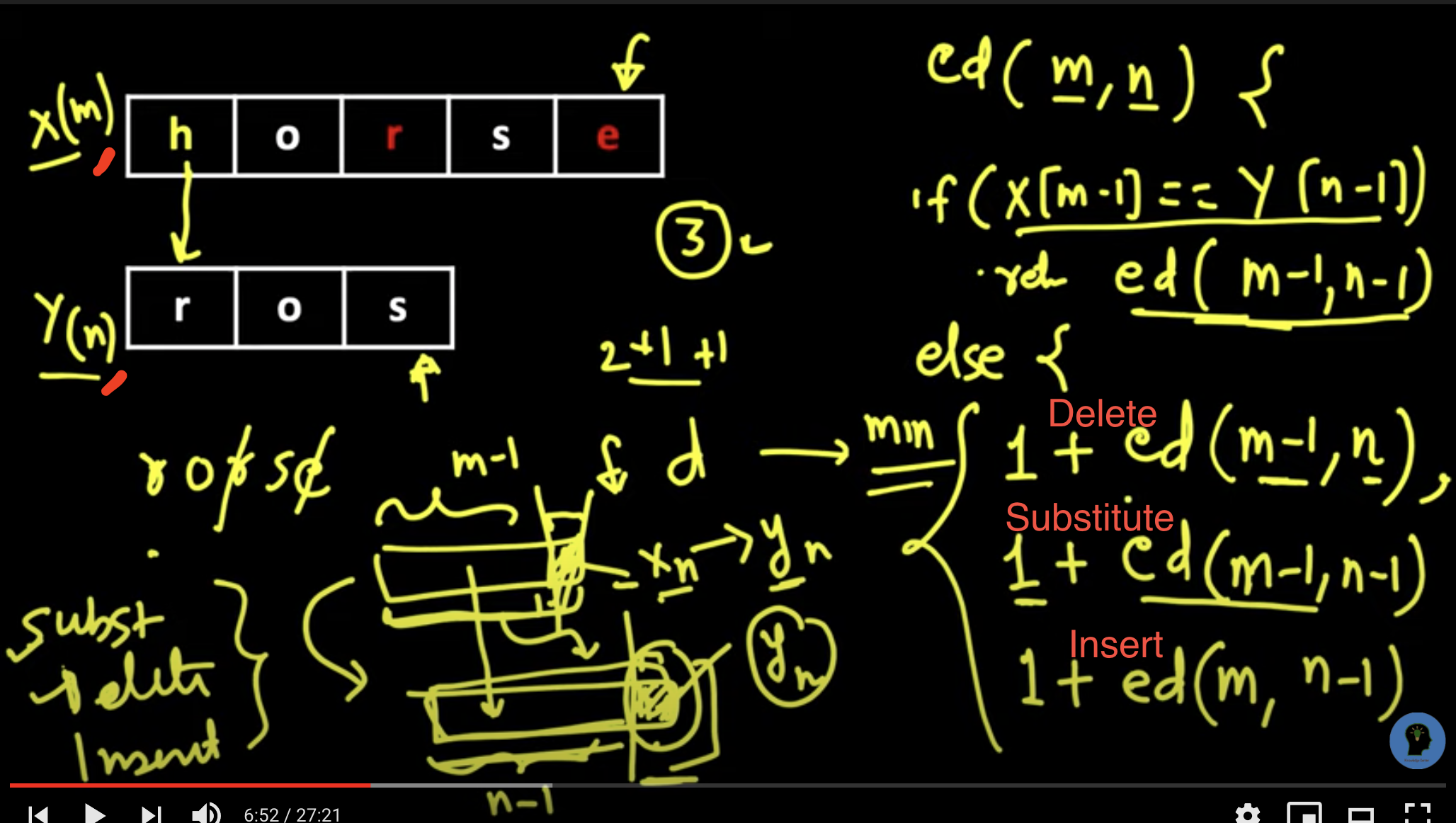class Solution:
def minDistanceHelper(self,  word1, word2, one, two, curr):
# # base cases
# end of both words
if one == len(word1) and two == len(word2):
return curr
# end of any word
if one == len(word1):
return curr + len(word2) - two
if two == len(word2):
return curr + len(word1) - one

# # operations required to convert word1 to word2
# no operation needed
if word1[one] == word2[two]:
return self.minDistanceHelper(word1, word2, one+1, two+1, curr)

# insert -> insert word2[two] into word1
insert = self.minDistanceHelper(word1, word2, one, two+1, curr+1)
# delete -> delete char at word1[one] (move to next char at word1)
delete = self.minDistanceHelper(word1, word2, one+1, two, curr+1)
# replace -> replace word1[one] with word2[two]
replace = self.minDistanceHelper(word1, word2, one+1, two+1, curr+1)

return min(insert, delete, replace)

def minDistance(self, word1, word2):
return self.minDistanceHelper(word1, word2, 0, 0, 0)


Brute-force that can work with caching

class Solution:
def minDistanceHelper(self,  word1, word2, one, two):
# # base cases
# end of both words
if one == len(word1) and two == len(word2):
return 0
# end of any word
if one == len(word1):
return len(word2) - two
if two == len(word2):
return len(word1) - one

# # operations required to convert word1 to word2
# no operation needed
if word1[one] == word2[two]:
return self.minDistanceHelper(word1, word2, one+1, two+1)

# insert -> insert word2[two] into word1
insert = self.minDistanceHelper(word1, word2, one, two+1)
# delete -> delete char at word1[one] (move to next char at word1)
delete = self.minDistanceHelper(word1, word2, one+1, two)
# replace -> replace word1[one] with word2[two]
replace = self.minDistanceHelper(word1, word2, one+1, two+1)
return 1 + min(insert, delete, replace)

def minDistance(self, word1, word2):
return self.minDistanceHelper(word1, word2, 0, 0)


### Memoization (Top Down Dynamic programming)

Improvement of the brute-force solution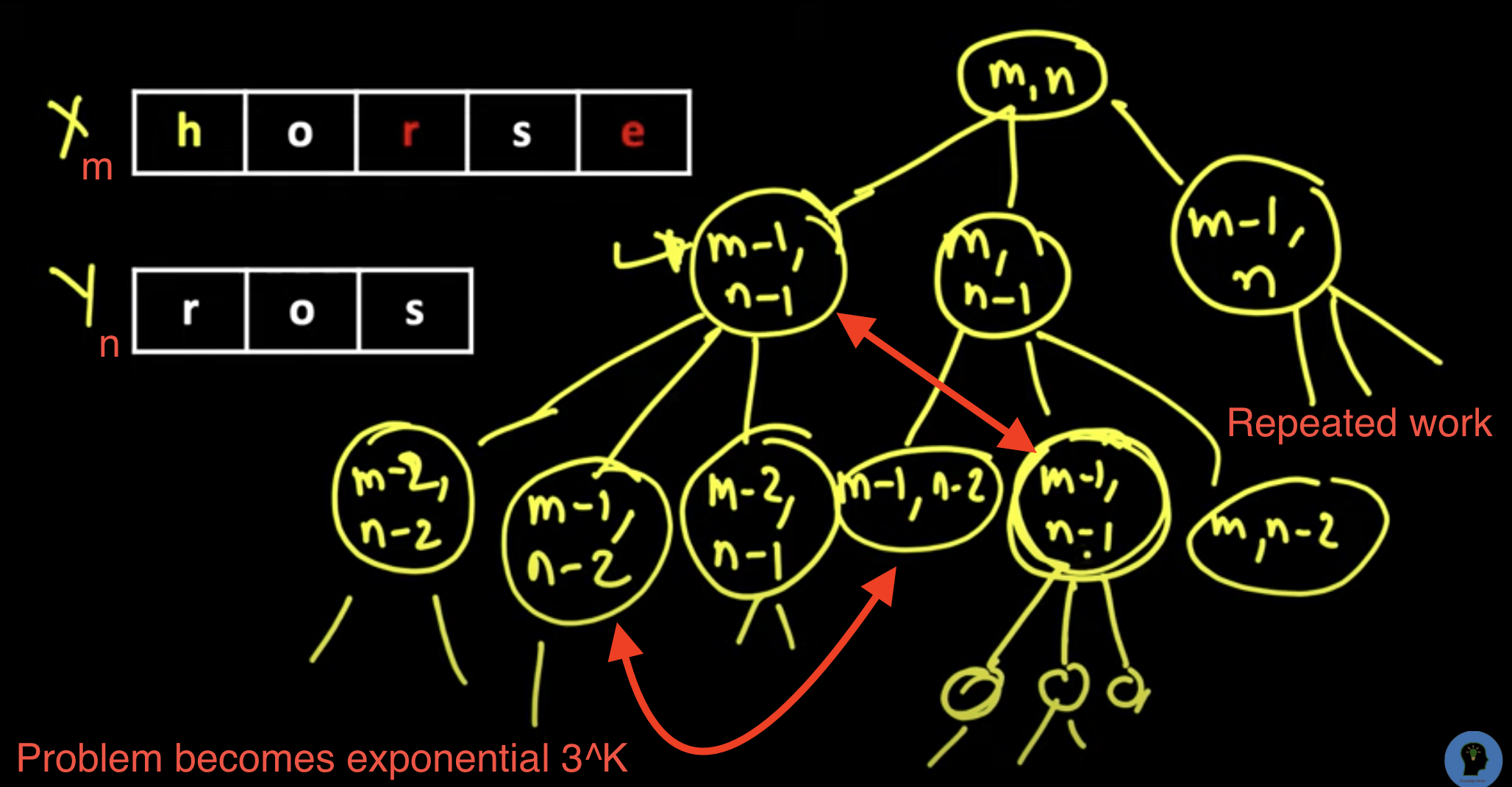class Solution:
def minDistanceHelper(self, dp, word1, word2, one, two):
# # base cases
# end of both words
if one == len(word1) and two == len(word2):
return 0
# end of any word
if one == len(word1):
return len(word2) - two
if two == len(word2):
return len(word1) - one

# # check cache
if dp[one][two]:
return dp[one][two]

# # operations required to convert word1 to word2
# no operation needed
if word1[one] == word2[two]:
dp[one][two] = self.minDistanceHelper(dp, word1, word2, one+1, two+1)
else:
# insert -> insert word2[two] into word1
insert = self.minDistanceHelper(dp, word1, word2, one, two+1)
# delete -> delete char at word1[one] (move to next char at word1)
delete = self.minDistanceHelper(dp, word1, word2, one+1, two)
# replace -> replace word1[one] with word2[two]
replace = self.minDistanceHelper(dp, word1, word2, one+1, two+1)
dp[one][two] = 1 + min(insert, delete, replace)

return dp[one][two]

def minDistance(self, word1, word2):
dp = [[False for _ in range(len(word2)+1)] for _ in range(len(word1)+1)]
return self.minDistanceHelper(dp, word1, word2, 0, 0)


### Tabulation (Bottom Up Dynamic programming)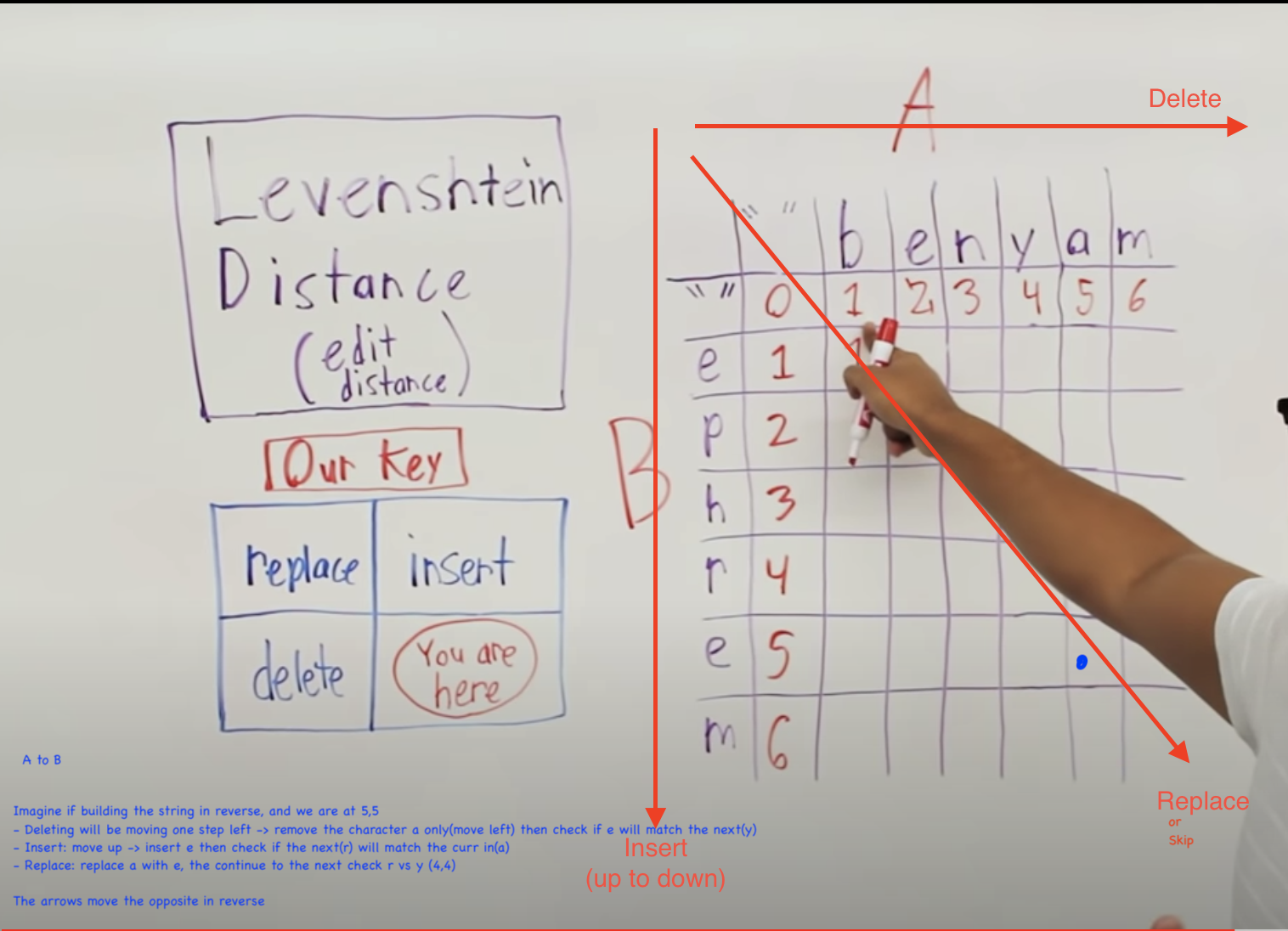arrows show how to get results of certain actions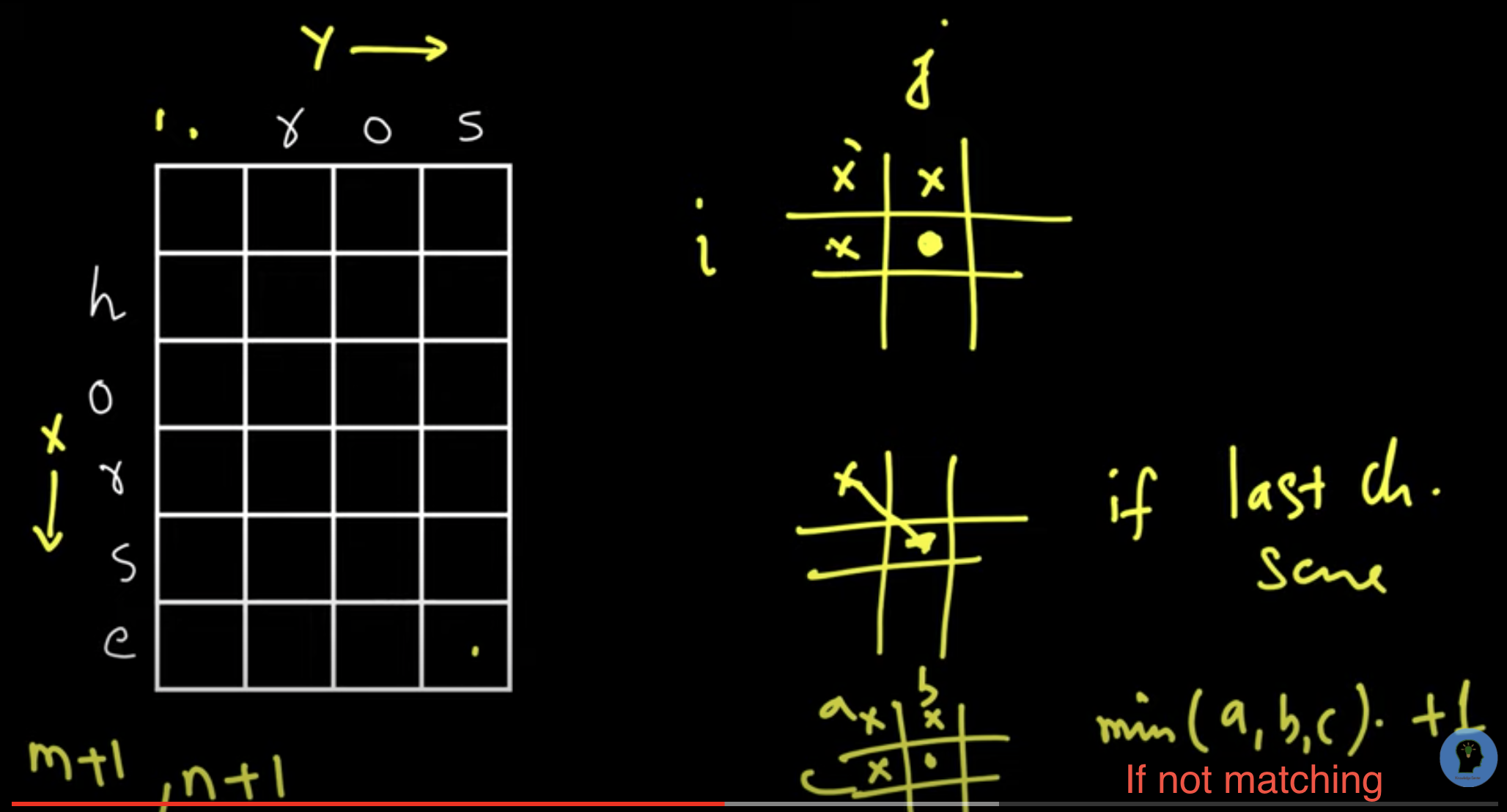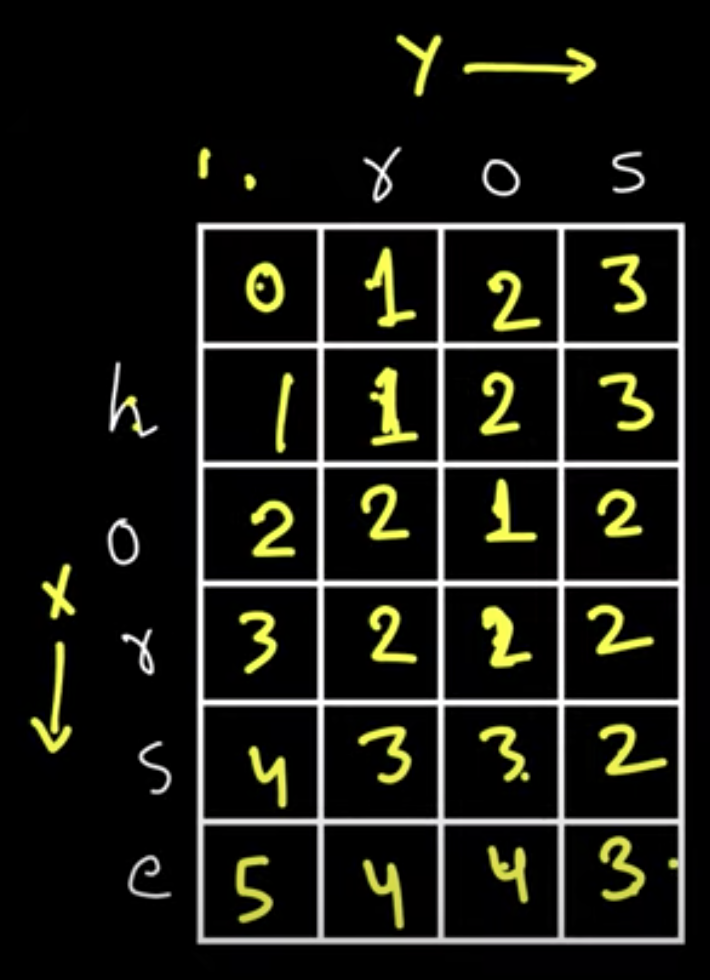x to y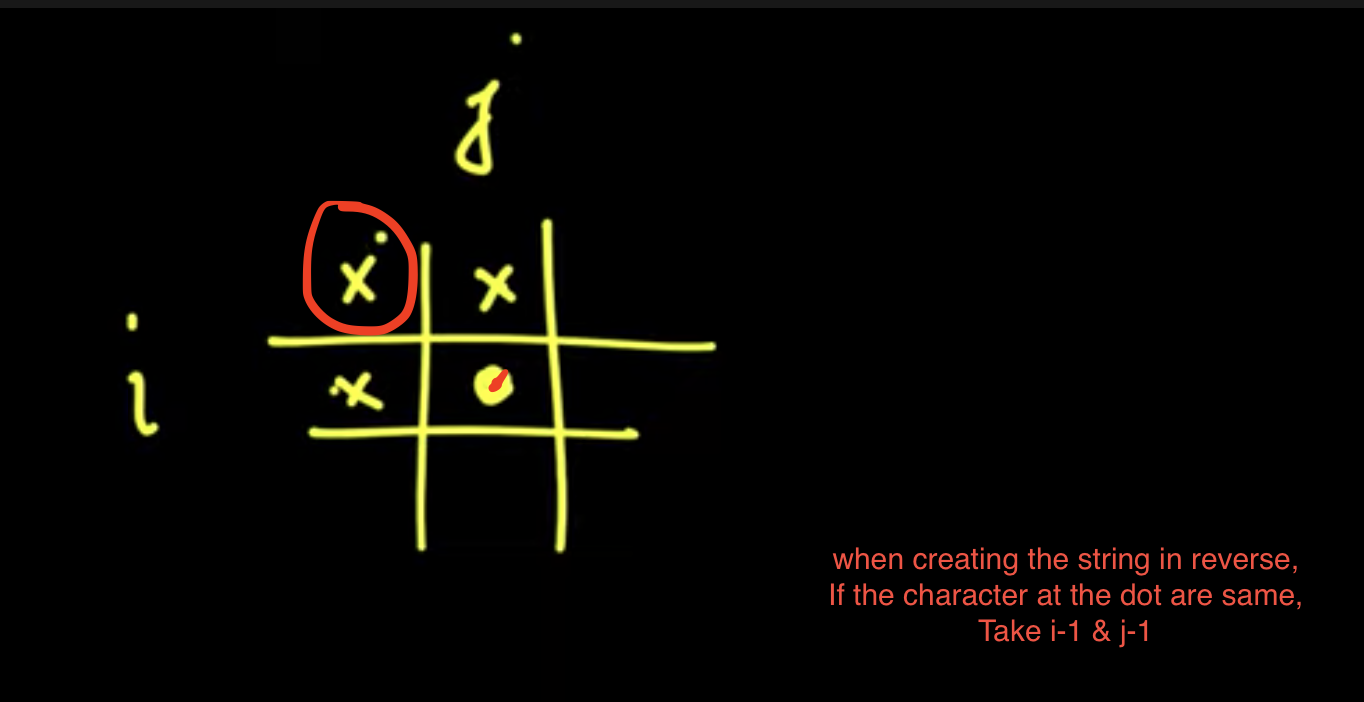class Solution:
def minDistance(self, word1, word2):

dp = [[0 for _ in range(len(word1)+1)] for _ in range(len(word2)+1)]

# # fill the values we know
for i in range(len(dp)):
dp[i] = i
for i in range(len(dp)):
dp[i] = i

for two in range(1, len(dp)):
for one in range(1, len(dp)):
left = dp[two][one-1]  # delete
top = dp[two-1][one]  # insert
diagonal = dp[two-1][one-1]  # replace/skip

if word1[one-1] == word2[two-1]:
dp[two][one] = dp[two-1][one-1]  # skip
else:
dp[two][one] = min(left, top, diagonal) + 1

return dp[-1][-1]